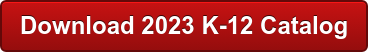<img alt="" src="https://secure.smart-enterprise-52.com/266730.png" style="display:none;">

## RobotLAB BlogWho doesn’t like seeing Optimus prime fight Megatron? Or one of the robots in “Pacific Rim” use an oil tanker to fight one of the monsters? Well controlling a robot, large or small, involves the use of cosines, among other mathematical equations. However, cosines, like quadratic equations and vectors, are hard to understand using just a math book. Unless your students are really interested in figuring out how tall buildings are, they may not care to learn how cosines factor into things like designing and building a roof. RobotsLAB solves this problem by stripping down the concept of cosines to their core functionality, giving the students something physical (and pretty cool) to look at and makes the concept easier to understand.In this lesson, Get the Cosines, the ARMBOT (a robotic arm controlled through the tablet) shows that the relationship between height, distance and angles is really a cosine equation: AB2+BC2–2+AB *BC*cosb. The Prepare tab is use to give the teacher and students and idea of how cosines traditionally work. Next, the Demonstrate tab is where the teacher can play with the values and come up with their own questions for the students. The students can be challenged to figure out either what angle they need or what distance is required to move the arm far enough to grab the block under the investigate tab. The entire time that the arm is moving there are three red dots on the robot that are used to represent the different angles A, B and C.Rather than drawing triangles on a dry erase board or using abstract examples like building height or the shade a tree gives, the ARMBOT and lesson show the students how a particular cosine problem works in a real, physical environment. Increasing or decreasing the distance changes the angle of the arm on the robot which is represented two ways:  the ARMBOT arm and the 3 red dots moving. The end result of the lesson is that students can understand how cosine equations work and are one step closer to learning how to fight apocalyptic monsters.